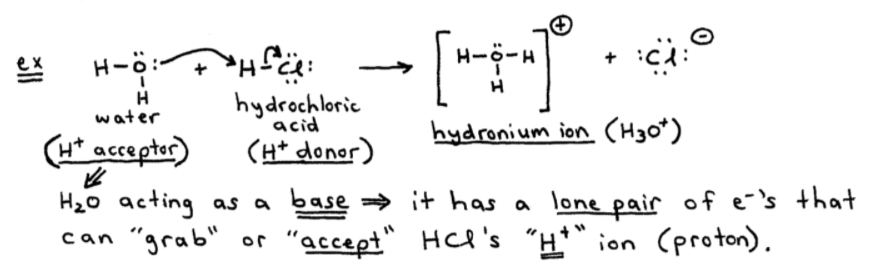# S14E1 - Bronsted-Lowry Acids and Bases, and Conjugate Acid-Base Pairs

## Acids and Bases

Acid-Base chemistry is important in a wide variety of applications.

ex:  acid rain, blood pH, aquariums, etc...

➞ acids - sour taste

➞ bases - alkalis, taste bitter, slippery (soap, Drano)

==========

## Arrhenius Acids and Bases

Arrhenius Acid  =  a substance that produces H+ ions (protons) in aqueous solutions.

Arrhenius Base  =  a substance that produces OH- ions in aqueous solutions.

The above two definitions are limited to only aqueous solutions.  The Bronsted-Lowry definitions, however, are not as limited...

==========

## Bronsted-Lowry Acids and Bases

Bronsted-Lowry Acid - a substance that is a proton (H+) donor.

Bronsted-Lowry Base - a substance that is a proton (H+) acceptor.

Check out the example below. You can see that H2O is acting as a Bronsted-Lowry base.

It has a lone pair of electrons that can "grab" or "accept" HCl's proton (H+ ion)...Now, I want to show you a typical acid-base reaction that ends up with products that can be labeled as a conjugate acid and a conjugate base.

Here are the definitions of each...

----------

### Conjugate Acid-Base Definitions

Conjugate Acid  =  formed when a proton (H+) is added to the base.

Conjugate Base  =  this is what's "left over" after the acid has its H+ (proton) removed.## Conjugate Acid-Base Pairs

NOTES - Here's 4 key points about the above generalized reaction.  The 3rd note is a bit lengthy.

1.  HA and A- are a conjugate acid-base pair.

2.  H2O and H3O+ are a conjugate acid-base pair.

3.  The above reaction is essentially a competition for the proton (H+) between the two bases (A- and H2O).

Here's the equilibrium expression for the above reaction:If H2O is a much stronger base than A-, then the equilibrium lies to the right (larger Ka).

Why? Because H2O is "better than" A- at "grabbing" or accepting a proton (H+) in the reaction.  On the other hand...

----------

If A- is a much stronger base than H2O, then the equilibrium lies to the left (smaller Ka).

Why? Because being the stronger base, A- is "better than" H2O at "grabbing" or accepting a proton (H+) in the reaction.

----------

4.  Because H2O(l) is not included in the equilibrium expression, the Ka has the same form as that for the simple dissociation reaction:

HA (aq)   ⇌   H(aq)   +   A(aq)

( but don't forget the role of H2O ).

==========

My next video post from SECTION 14 - Acids and Bases will cover Acid Strength and Conjugate Acid-Base Examples and Practice Problems.